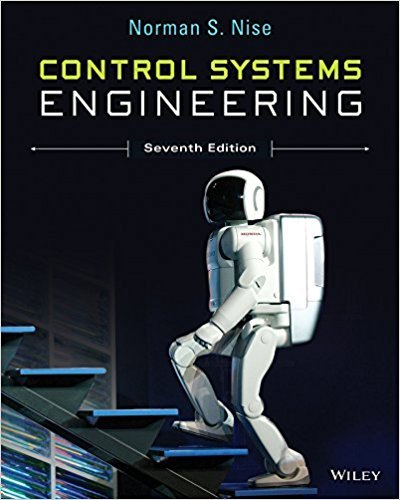×
Get Full Access to Control Systems Engineering - 7 Edition - Chapter 7 - Problem 56
Get Full Access to Control Systems Engineering - 7 Edition - Chapter 7 - Problem 56

×

# A simplified model of the steering of a four-wheel driveISBN: 9781118170519 162

## Solution for problem 56 Chapter 7

Control Systems Engineering | 7th Edition

• Textbook Solutions
• 2901 Step-by-step solutions solved by professors and subject experts
• Get 24/7 help from StudySoup virtual teaching assistantsControl Systems Engineering | 7th Edition

4 5 1 424 Reviews
19
3
Problem 56

A simplified model of the steering of a four-wheel drive vehicle is shown in Figure P7.26. Gf r* K Gr f u (r + + ) + e r FIGURE P7.26 Steering model for a four-wheel drive vehicle7 In this block diagram, the output r is the vehicles yaw rate, while f and r are the steering angles of the front and rear tires, respectively. In this model, r*s s 300 0:8 s 10 1 ; Gf s h1s h2 s2 a1s a2 Grs h3s b1 s2 a1s a2 and K(s) is a controller to be designed. (Yin, 2007). a. Assuming a step input for f , find the minimum system type of the controller K(s) necessary so that in steady-state the error, as defined by the signal e in Figure P7.26, is zero if at all possible. b. Assuming a step input for f , find the system type of the controller K(s) necessary so that in steady state the error as defined by f r is zero if at all possible.

Step-by-Step Solution:
Step 1 of 3

Step 2 of 3

Step 3 of 3

##### ISBN: 9781118170519

This full solution covers the following key subjects: figure, model, steering, controller, Zero. This expansive textbook survival guide covers 13 chapters, and 734 solutions. Control Systems Engineering was written by and is associated to the ISBN: 9781118170519. The answer to “A simplified model of the steering of a four-wheel drive vehicle is shown in Figure P7.26. Gf r* K Gr f u (r + + ) + e r FIGURE P7.26 Steering model for a four-wheel drive vehicle7 In this block diagram, the output r is the vehicles yaw rate, while f and r are the steering angles of the front and rear tires, respectively. In this model, r*s s 300 0:8 s 10 1 ; Gf s h1s h2 s2 a1s a2 Grs h3s b1 s2 a1s a2 and K(s) is a controller to be designed. (Yin, 2007). a. Assuming a step input for f , find the minimum system type of the controller K(s) necessary so that in steady-state the error, as defined by the signal e in Figure P7.26, is zero if at all possible. b. Assuming a step input for f , find the system type of the controller K(s) necessary so that in steady state the error as defined by f r is zero if at all possible.” is broken down into a number of easy to follow steps, and 173 words. The full step-by-step solution to problem: 56 from chapter: 7 was answered by , our top Engineering and Tech solution expert on 11/23/17, 05:05AM. Since the solution to 56 from 7 chapter was answered, more than 288 students have viewed the full step-by-step answer. This textbook survival guide was created for the textbook: Control Systems Engineering, edition: 7.

Unlock Textbook Solution